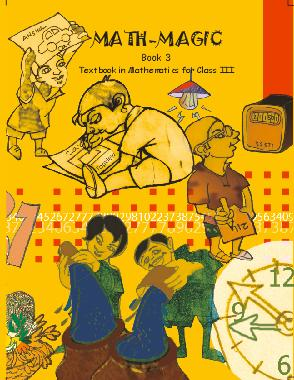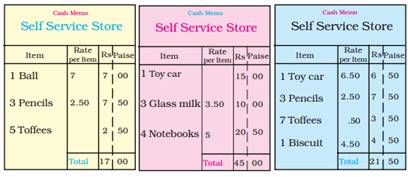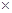# NCERT Solutions for Class 3 Maths Rupees and Paise

NCERT solutions for Class 3 Mathematics## NCERT Solutions for Class 3 Maths Rupees and Paise

CHAPTER 14 Class 3 Maths Rupees and Paise

Ans. Rs. 22.

Ans. Rs. 10.

Ans. Rs. 7.50.

Ans. Rs. 15.50.

Ans. Rs. 9.

Ans. Rs. 12.

Ans. Rs. 8.50.

### 8. What can you buy if you have a twenty-rupee note?

Ans. 1 toy car, 1 lemon juice, 1 banana.

1 ball, 1 doll, 1 notebook.

1 toy car, 1 banana, 1 glass milk.

1 toy car, 1 packet of biscuit, 1 toffee.

1 toy car, 1 toy giraffe, 1 glass milk.

### 9. Monu prepared the following cash memos:

Check the cash memos and correct them if you find a mistake.Ans. Corrected cash memo are given below:

 Cash MemoSelf Service Store Item Rate Rs Paise 1 Ball 7 7 00 2 Pencil 2.50 5 00 5 Toffees 1.50 2 50 Total 14 50
 Cash MemoSelf Service Store Item Rate Rs Paise 1 Toy car 15 15 00 3 Glass milk 3.50 10 50 4 Notebooks 5 20 00 Total 45 50
 Cash MemoSelf Service Store Item Rate Rs Paise 1 Toy car 15 15 00 3 Pencils 2.50 7 50 7 Toffees 0.50 3 50 1 Biscuits 4.50 4 50 Total 30 50

(a)

 Rs. 12. 50 Rs. +13. 00 Rs.

Ans.

 Rs. 12 50 Rs. 13 00 Rs. 25 50

(b)

 Rs. 55. 50 Rs. +14. 00 Rs.

Ans.

 Rs. 55 50 Rs. 14 00 Rs. 69 50

(c)

 Rs. 30. 00 Rs. +31. 50 Rs.

Ans.

 Rs. 30 00 Rs. 31 50 Rs. 61 50

### 11. Subtract the following:

(a)

 Rs. 15. 50 Rs. -11. 50 Rs.

Ans.

 Rs. 15 50 Rs. -11 50 Rs. 14 00

(b)

 Rs. 103. 50 Rs. -62. 00 Rs.

Ans.

 Rs. 103 50 Rs. -62 00 Rs. 41 50

(c)

 Rs. 19. 50 Rs. – 7. 00 Rs.

Ans.

 Rs. 19. 50 Rs. – 7. 00 Rs. 12. 50

### 12. You have 30 rupees with you. Find out how much money will be left after buying the following items: One ball, one doll and one toy giraffe. Total cost of Rs. 21.50. Money left Rs. 8.50.

Ans. Cost of 1 ball = Rs. 7

Cost of 1 doll = Rs. 8

Cost of 1 toy giraffe = Rs. 6.50

So, Total cost = Rs. 7 + Rs. 8 + Rs. 6.50 = Rs. 21.50

Money left = Total Money – Money Spent = Rs. 30 – Rs. 12.50 = Rs. 8.50.

### 13. Two bananas, one pack of biscuits and two glasses of lemon juice. Total cost Rs. 13.50. Money left Rs. 16.50.

Ans. Cost of 2 bananas = Rs. 1.502 = Rs. 3

Cost of 1 pack of biscuits = RS. 4.50

Cost of 2 glasses of lemon juice = Rs. 32 = Rs. 6

So, Total cost = Rs. 3 + Rs. 4.50 + Rs. 6 = Rs. 13.50

Money left = Total money – Money spent

= Rs. 30 – Rs. 13.50 = Rs. 16.50.

### 14. Three notebooks, two pencils and two erasers. Total cost Rs. 22. Money left Rs. 8.

Ans. Cost of 3 notebooks = Rs. 53 = Rs. 15

Cost of 2 pencils = Rs. 2.502 = Rs. 5

Cost of 2 erasers = Rs. 12 = Rs. 2

Total cost = Rs. 15 + Rs. 5 + Rs. 2 = Rs. 22

Money left = Total money – Money spent = Rs. 30 – Rs. 22 = Rs. 8

### 15. Three friends wanted to buy a cricket bat and ball. Bina had Rs. 48.50, Raman had Rs. 55. 50 and Venu had Rs. 38.00. How much money did they have in all?

Ans. Money with Bina = Rs. 48.50

Money with Raman = Rs. 55.50

Money with Venu = Rs. 38.00

Total money = Rs. 142.00

### 16. Hari booked a railway ticket for Rs. 62.50. He gave a 100 rupee note. How much money will he get back with the ticket?

Ans. Total money with Hari = Rs. 100

Money spent on ticket = Rs. 62.50

Money left = Rs. 100 – Rs. 62.50

= Rs. 37.50

### 17. Gita and her friends went shopping. She bought things for Rs. 58, Rs. 37 and Rs. 22. Gita had a hundred-rupee note. How much money should she borrow from her friends to pay the bill?

Ans. Total money with Geeta = Rs. 100

Money spent by Geeta = Rs. 58 + Rs. 37 + Rs. 22 = Rs. 117

Money to be borrowed = Rs. 117 – Rs. 110 = Rs. 17.

Class 3 Maths Rupees and Paise

### 18. Find the cost of tickets:

(a) Bhupen is going from New Jalpaiguri to Alipurduar. That is the cost of his ticket?

Ans.  Cost of ticket from New Jalpaiguri to Alipurduar is Rs. 28.00.

(b) Indira has to go from New Jalpaiguri to Goalpara. How much does she pay for the ticket?

Ans.  Cost of ticket from New Jalpaiguri to Goalpara is Rs. 49.50.

(c) Debu, Shoma and Gobind are going from New Jalpaiguri to New Mal. What amount will they pay for tickets?

Ans. Fare from New Jalpaiguri to New Mal = Rs. 12. 50

Amount of fare from debu, Shoma and Gobind = Rs. 12. 503 = Rs. 37.50

Money given for tickets = Rs. 50

Money spent on tickets = Rs. 37.50

Money got back = Rs. 50 – Rs. 37.50 = Rs. 12.50.

## NCERT solutions for Class 3 Mathematics Chapter 14 Rupees and Paise

NCERT Solutions Class 3 Maths Rupees and Paise PDF (Download) Free from myCBSEguide app and myCBSEguide website. Ncert solution class 3 Maths includes text book solutions from Class 3 Maths Book . NCERT Solutions for CBSE Class  Maths have total 14 chapters. 3 Maths NCERT Solutions in PDF for free Download on our website. Ncert Maths class 3 solutions PDF and solutions with latest modifications and as per the latest CBSE syllabus are only available in myCBSEguide.

## CBSE app for Students

To download NCERT Solutions for Class 3 EVS Hindi English, Maths do check myCBSEguide app or website. myCBSEguide provides sample papers with solution, test papers for chapter-wise practice, NCERT solutions, NCERT Exemplar solutions, quick revision notes for ready reference, CBSE guess papers and CBSE important question papers. Sample Paper all are made available through the best app for CBSE students and myCBSEguide website.### Test Generator

Create question paper PDF and online tests with your own name & logo in minutes.### myCBSEguide

Question Bank, Mock Tests, Exam Papers, NCERT Solutions, Sample Papers, Notes